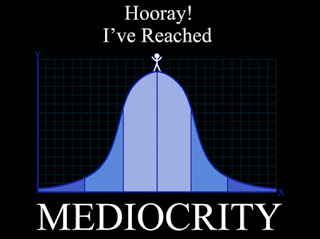Graph is Bell Curve. Why?What is the value of positive integer $n$ such that $\large { 1000 - n \choose n}$ is maximized?

×